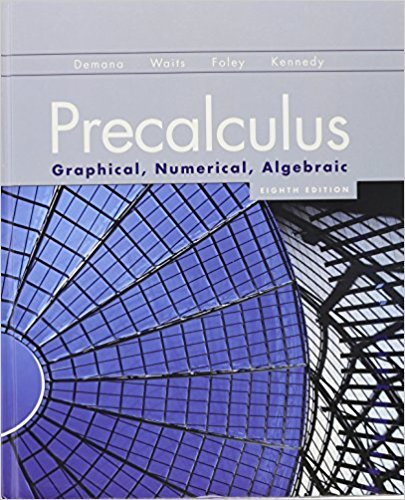×
×

# Solutions for Chapter 10: An Introduction to Calculus: Limits, Derivatives, and Integrals## Full solutions for Precalculus: Graphical, Numerical, Algebraic | 8th Edition

ISBN: 9780321656933Solutions for Chapter 10: An Introduction to Calculus: Limits, Derivatives, and Integrals

Solutions for Chapter 10
4 5 0 322 Reviews
28
5
##### ISBN: 9780321656933

This expansive textbook survival guide covers the following chapters and their solutions. Chapter 10: An Introduction to Calculus: Limits, Derivatives, and Integrals includes 45 full step-by-step solutions. This textbook survival guide was created for the textbook: Precalculus: Graphical, Numerical, Algebraic, edition: 8th Edition. Since 45 problems in chapter 10: An Introduction to Calculus: Limits, Derivatives, and Integrals have been answered, more than 43534 students have viewed full step-by-step solutions from this chapter. Precalculus: Graphical, Numerical, Algebraic was written by and is associated to the ISBN: 9780321656933.

Key Calculus Terms and definitions covered in this textbook
• Algebraic model

An equation that relates variable quantities associated with phenomena being studied

• Angle

Union of two rays with a common endpoint (the vertex). The beginning ray (the initial side) can be rotated about its endpoint to obtain the final position (the terminal side)

• Annuity

A sequence of equal periodic payments.

• Augmented matrix

A matrix that represents a system of equations.

• Back-to-back stemplot

A stemplot with leaves on either side used to compare two distributions.

• Degree

Unit of measurement (represented by the symbol ) for angles or arcs, equal to 1/360 of a complete revolution

• Derivative of ƒ at x a

ƒ'(a) = lim x:a ƒ(x) - ƒ(a) x - a provided the limit exists

• Direction of an arrow

The angle the arrow makes with the positive x-axis

• Elimination method

A method of solving a system of linear equations

• Factor

In algebra, a quantity being multiplied in a product. In statistics, a potential explanatory variable under study in an experiment, .

• Integers

The numbers . . ., -3, -2, -1, 0,1,2,...2

• Line of travel

The path along which an object travels

• Mean (of a set of data)

The sum of all the data divided by the total number of items

• n factorial

For any positive integer n, n factorial is n! = n.(n - 1) . (n - 2) .... .3.2.1; zero factorial is 0! = 1

• Origin

The number zero on a number line, or the point where the x- and y-axes cross in the Cartesian coordinate system, or the point where the x-, y-, and z-axes cross in Cartesian three-dimensional space

• Partial sums

See Sequence of partial sums.

• Positive linear correlation

See Linear correlation.

• Solve algebraically

Use an algebraic method, including paper and pencil manipulation and obvious mental work, with no calculator or grapher use. When appropriate, the final exact solution may be approximated by a calculator

• Summation notation

The series a nk=1ak, where n is a natural number ( or ?) is in summation notation and is read "the sum of ak from k = 1 to n(or infinity).” k is the index of summation, and ak is the kth term of the series

• Window dimensions

The restrictions on x and y that specify a viewing window. See Viewing window.

×